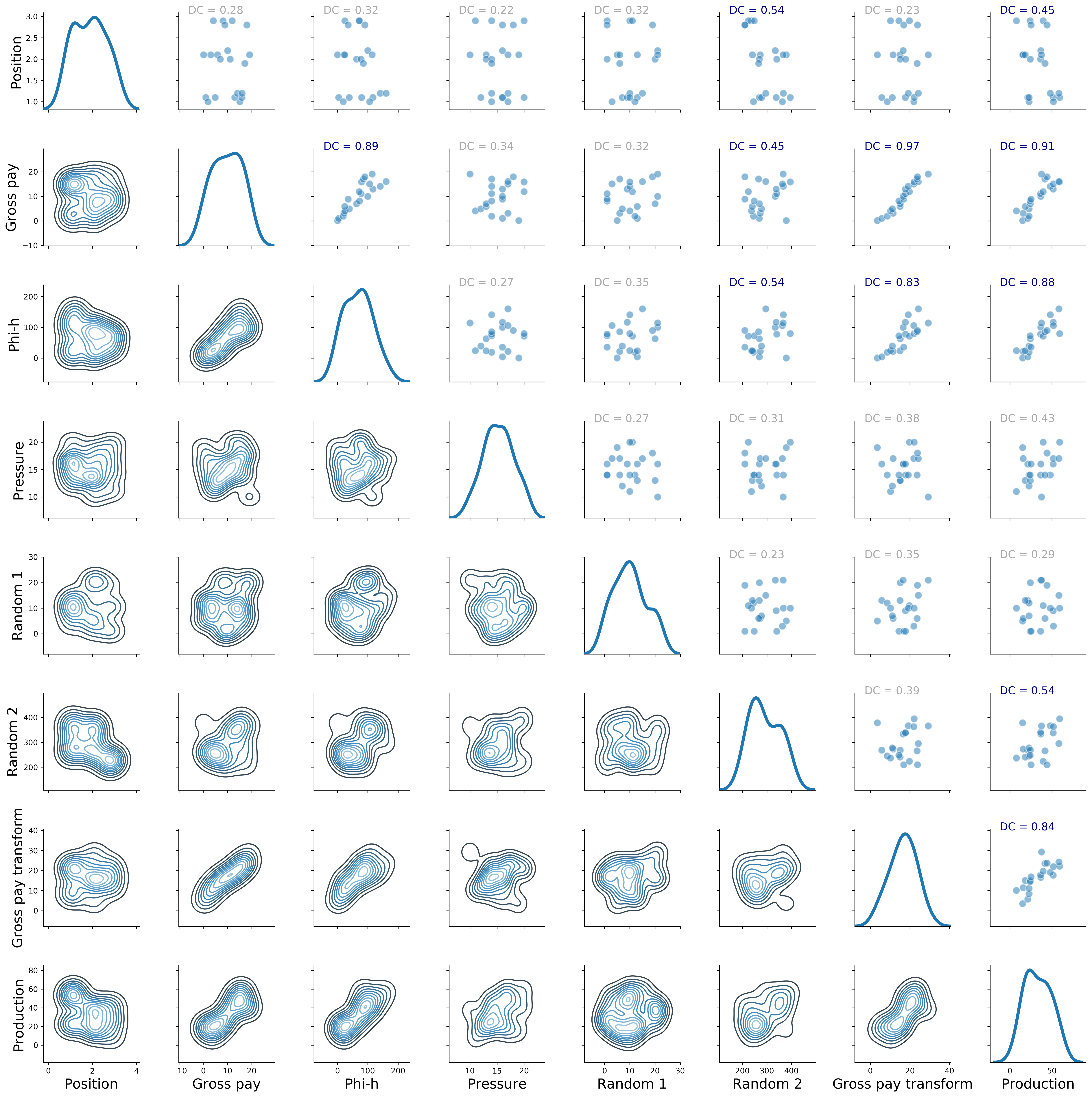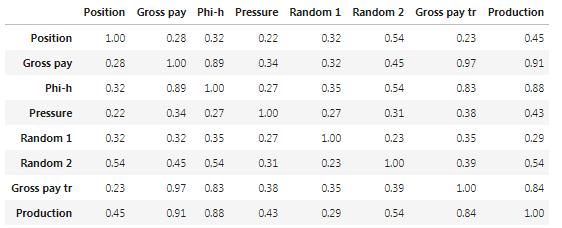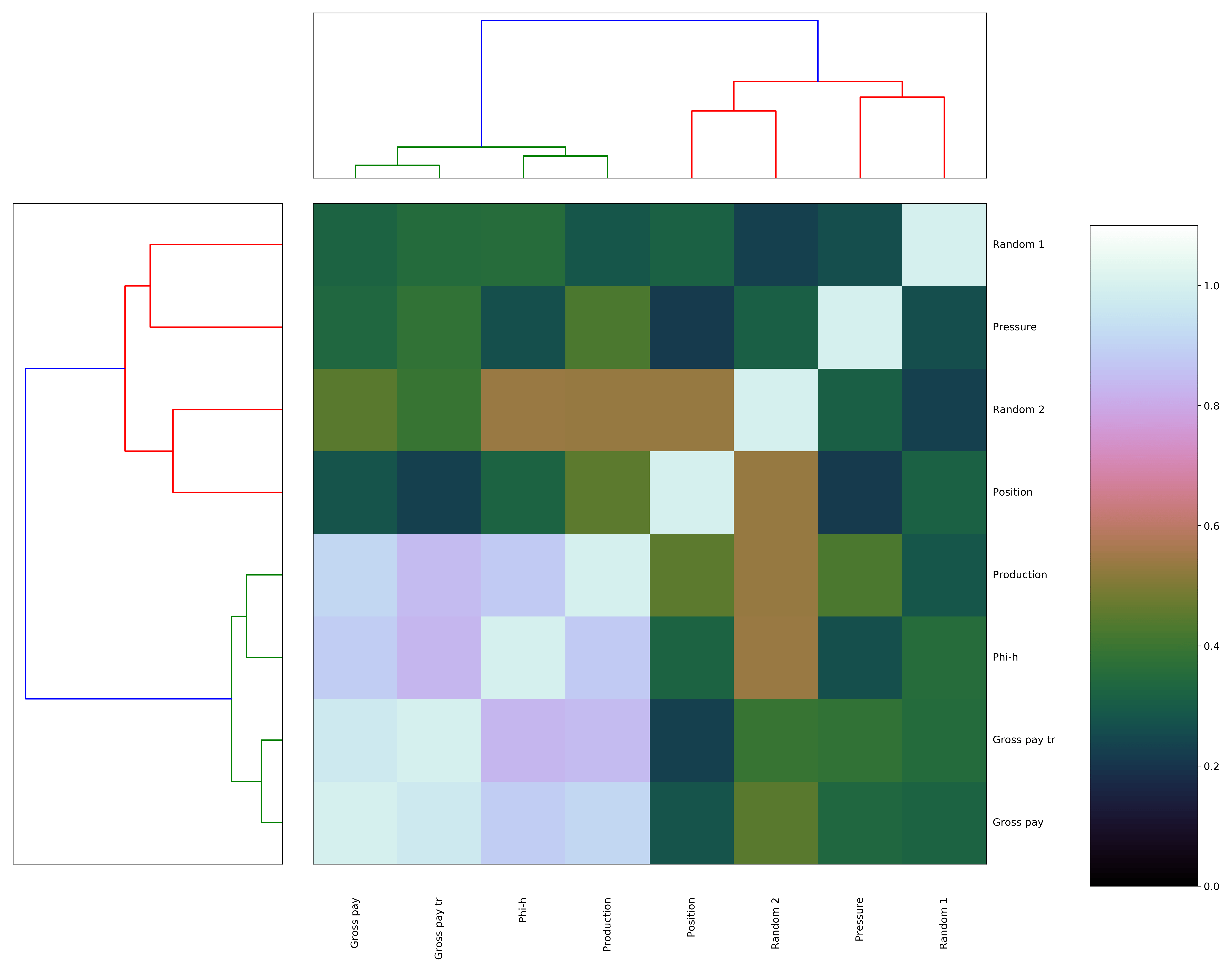# Data exploration in Python: distance correlation and variable clustering

April 10, 2019

In my last post I wrote about visual data exploration with a focus on correlation, confidence, and spuriousness. As a reminder to aficionados, but mostly for new readers’ benefit: I am using a very small toy dataset (only 21 observations) from the paper Many correlation coefficients, null hypotheses, and high value (Hunt, 2013).

The dependent/target variable is oil production (measured in tens of barrels of oil per day) from a marine barrier sand. The independent variables are: Gross pay, in meters; Phi-h, porosity multiplied by thickness, with a 3% porosity cut-off; Position within the reservoir (a ranked variable, with 1.0 representing the uppermost geological facies, 2.0 the middle one, 3.0 the lowest one); Pressure draw-down in MPa. Three additional ‘special’ variables are: Random 1 and Random 2, which are range bound and random, and were included in the paper, and Gross pay transform, which I created specifically for this exercise to be highly correlated to Gross pay, by passing Gross pay to a logarithmic function, and then adding a bit of normally distributed random noise.

##### Correlation matrix with ellipses

I am very pleased with having been able to put together, by the end of it, a good looking scatter matrix that incorporated:

• bivariate scatter-plots in the upper triangle, annotated with rank correlation coefficient, confidence interval, and probability of spurious correlation
• contours in the lower triangle
• shape of the bivariate distributions (KDE) on the diagonal

In a comment to the post, Matt Hall got me thinking about other ways to visualize the correlation coefficient.  I did not end up using a colourmap for the facecolour of the plot (although this would probably be relatively easy, in an earlier attempt using hex-bin plots, the colourmap scaling of each plot independently – to account for outliers – proved challenging). But after some digging I found the Biokit library, which comes with a lot of useful visualizations, among which `corrplot` is exactly what I was looking for. With only a bit of tinkering I was able to produce, shown in Figure 1, a correlation matrix with:

• correlation coefficient in upper triangle (colour and intensity indicate whether positive or negative correlation, and its strength, respectively)
• bivariate ellipses in the lower triangle (ellipse direction and colour indicates whether positive or negative correlation; ellipticity and colour intensity are proportional to the correlation coefficient)Figure 1. Correlation matrix using the Biokit library

Also notice that – quite conveniently – the correlation matrix of Figure 1 is reordered with strongly correlated variables adjacent to one another, which facilitates interpretation. This is done using the rank correlation coefficient, with `pandas.DataFrame.corr`, and Biokit’s `corrplot`:

```corr = data.corr(method='spearman')
c = corrplot.Corrplot(corr)
c.plot(method='ellipse', cmap='PRGn_r', shrink=1, rotation=45, upper='text', lower='ellipse')
fig = plt.gcf()
fig.set_size_inches(10, 8);```

The insightful take-away is that with this reordering, the more ‘interesting’ variables, because of strong correlation (as defined in this case by the rank correlation coefficient), are close together and reposition along the diagonal, so we can immediately appreciate that Production, Phi-h, and Gross Pay, plus to a lesser extent position (albeit this one with negative correlation to production) are related to one another. This is a great intuition, and supports up our hypothesis (in an inferential test), backed by physical expectation, that production should be related to those other quantities.

But I think it is time to move away from either Pearson or Spearman correlation coefficient.

##### Correlation matrix with distance correlation and its p-value

I learned about distance correlation from Thomas when we were starting to work on our 2018 CSEG/CASP Geoconvention talk Data science tools for petroleum exploration and production“. What I immediately liked about distance correlation is that it does not assume a linear relationship between variables, and even more importantly, whereas with Pearson and Spearman a correlation value of zero does not prove independence between any two variables, a distance correlation of zero does mean that there is no dependence between those two variables.

Working on the R side, Thomas used the Energy inferential statistic package. With the`dcor` function he calculated the distance correlation, and with the `dcov.test `function the p-value via bootstrapping.

For Python, I used the `dcor` and `dcor.independence.distance_covariance_test` from the dcor library (with many thanks to Carlos Ramos Carreño, author of the Python library, who was kind enough to point me to the table of energy-dcor equivalents). So, for example, for one variable pair, we can do this:

```print ("distance correlation = {:.2f}".format(dcor.distance_correlation(data['Production'],
data['Gross pay'])))
print("p-value = {:.7f}".format(dcor.independence.distance_covariance_test(data['Production'],
data['Gross pay'],
exponent=1.0,
num_resamples=2000)))
>>> distance correlation  = 0.91
>>> p-value = 0.0004998```

So, wanting to apply these tests in a pairwise fashion to all variables, I modified the `dist_corr` function and `corrfunc` function from the existing notebook

```def dist_corr(X, Y, pval=True, nruns=2000):
""" Distance correlation with p-value from bootstrapping
"""
dc = dcor.distance_correlation(X, Y)
pv = dcor.independence.distance_covariance_test(X, Y, exponent=1.0, num_resamples=nruns)
if pval:
return (dc, pv)
else:
return dc```
```def corrfunc(x, y, **kws):
d, p = dist_corr(x,y)
#print("{:.4f}".format(d), "{:.4f}".format(p))
if p > 0.1:
pclr = 'Darkgray'
else:
pclr= 'Darkblue'
ax = plt.gca()
ax.annotate("DC = {:.2f}".format(d), xy=(.1, 0.99), xycoords=ax.transAxes, color = pclr, fontsize = 14)```

so that I can then just do:

```g = sns.PairGrid(data, diag_sharey=False)
axes = g.axes
g.map_upper(plt.scatter, linewidths=1, edgecolor="w", s=90, alpha = 0.5)
g.map_upper(corrfunc)
g.map_diag(sns.kdeplot, lw = 4, legend=False)
g.map_lower(sns.kdeplot, cmap="Blues_d")
plt.show();```Figure 2. Revised Seaborn pairgrid matrix with distance correlation colored by p-value (gray if p > 0.10, blue if p <= 0.10)

##### Clustering using distance correlation

I really like the result in Figure 2.  However, I want to have more control on how the pairwise plots are arranged; a bit like in Figure 1, but using my metric of choice, which would be again the distance correlation. To do that, I will first show how to create a square matrix of distance correlation values, then I will look at clustering of the variables; but rather than passing the raw data to the algorithm, I will pass the distance correlation matrix. Get ready for a ride!

For the first part, making the square matrix of distance correlation values, I adapted the code from this brilliant SO answer on Euclidean distance (I recommend you read the whole answer):

```# Create the distance method using distance_correlation
distcorr = lambda column1, column2: dcor.distance_correlation(column1, column2)
# Apply the distance method pairwise to every column
rslt = data.apply(lambda col1: data.apply(lambda col2: distcorr(col1, col2)))
# check output
pd.options.display.float_format = '{:,.2f}'.format
rslt
```Table I. Distance correlation matrix.

The matrix in Table I looks like what I wanted, but let’s calculate a couple of values directly, to be sure:

```print ("distance correlation = {:.2f}".format(dcor.distance_correlation(data['Production'],
data['Phi-h'])))
print ("distance correlation = {:.2f}".format(dcor.distance_correlation(data['Production'],
data['Position'])))```
```>>> distance correlation = 0.88
>>> distance correlation = 0.45```

Excellent, it checks!

Now I am going to take a bit of a detour, and use that matrix, rather than the raw data, to cluster the variables, and then display the result with a heat-map and accompanying dendrograms. That can be done with Biokit’s `heatmap`:

```data.rename(index=str, columns={"Gross pay transform": "Gross pay tr"}, inplace=True)
distcorr = lambda column1, column2: dcor.distance_correlation(column1, column2)
rslt = data.apply(lambda col1: data.apply(lambda col2: distcorr(col1, col2)))
h = heatmap.Heatmap(rslt)
h.plot(vmin=0.0, vmax=1.1, cmap='cubehelix')
fig = plt.gcf()
fig.set_size_inches(22, 18)
plt.gcf().get_axes().invert_xaxis();
```Figure 3. Biokit heatmap with dendrograms, using correlation distance matrix

That’s very nice, but please notice how much ‘massaging’ it took: first, I had to flip the axis for the dendrogram for the rows (on the left) because it would be incorrectly reversed by default; and then, I had to shorten the name of Gross-pay transform so that its row label would not end up under the colorbar (also, the diagonal is flipped upside down, and I could not reverse it or else the colum labels would go under the top dendrogram). I suppose the latter too could be done on the Matplotlib side, but why bother when we can get it all done much more easily with Seaborn? Plus, you will see below that I actually have to… but I’m putting the cart before the horses…. here’s the Seaborn code:

```g = sns.clustermap(rslt, cmap="mako",  standard_scale =1)
fig = plt.gcf()
fig.set_size_inches(18, 18);```

and the result in Figure 4. We really get everything for free!Figure 4. Seaborn clustermap, using correlation distance matrix

Before moving to the final fireworks, a bit of interpretation: again what comes up is that Production, Phi-h, Gross Pay, and Gross pay transform group together, as in Figure 1, but now the observation is based on a much more robust metric. Position is not as ‘close’, it is ends up in a different cluster, although its distance correlation from Production is 0.45, and the p-value is <0.10, hence it is still a relevant variable.

I think this is as far as I would go with interpretation. It does also show me that Gross pay, and Gross pay transform are very much related to one another, with high dependence, but it does still not tell me, in the context of variable selection for predicting Production, which one I should drop: I only know it should be Gross pay transform because I created it myself. For proper variable selection I will look at techniques like Least Absolute Shrinkage and Selection Operator (LASSO, which Thomas has showcased in his R notebook) and Recursive Feature Elimination (I’ll be testing Sebastan Raschka‘s Sequential Feature Selector from the `mlxtend` library).

##### Correlation matrix with distance correlation, p-value, and plots rearranged by clustering

I started this whole dash by saying I wanted to control how the pairwise plots were arranged in the scatter matrix, and that to do so required use of  Seaborn. Indeed, it turns out the reordered row/column indices (in our case they are the same) can be easily accessed:

```a = (g.dendrogram_col.reordered_ind)
print(a)
>>> [1, 6, 2, 7, 0, 5, 3, 4]```

and if this is the order of variables in the original DataFrame:

```b = list(data)
print (b)
>>> [Position', 'Gross pay', 'Phi-h', 'Pressure', 'Random 1', 'Random 2', 'Gross pay transform', 'Production']```

we can rearrange them with those reordered column indices:

```data = data[[b[i] for i in a]]
print(list(data))
>>> ['Gross pay', 'Gross pay transform', 'Phi-h', 'Production', 'Position', 'Random 2', 'Pressure', 'Random 1']```

after which we can simply re-run the code below!!!

```g = sns.PairGrid(data, diag_sharey=False)
axes = g.axes
g.map_upper(plt.scatter, linewidths=1, edgecolor="w", s=90, alpha = 0.5)
g.map_upper(corrfunc)
g.map_diag(sns.kdeplot, lw = 4, legend=False)
g.map_lower(sns.kdeplot, cmap="Blues_d")
plt.show()
```Figure 5. Revised Seaborn pairgrid matrix with distance correlation colored by p-value (gray if p > 0.10, blue if p <= 0.10), and plots rearranged by clustering results

## 8 thoughts on “Data exploration in Python: distance correlation and variable clustering”

1.David M. Baker on said:

Matteo, nice work! Is the code for “Correlation matrix with distance correlation, p-value, and plots rearranged by clustering” a bit off? When you rearrange the columns you refer to variable “a” and then the rearranged list does not look rearranged. David

•matteomycarta on said:

Hi David, thanks for the feedback

And you are spot on about the not-reordered list of variables!!
That’s what happens when you overwrite DataFrames, then run some code cells again… ha ha!
I updated the code in the post, and also just uploaded the notebook. Matteo

2.David M. Baker on said:

a = g.dendrogram_col.reordered_ind

•matteomycarta on said:

darn
I have to stop including code n the posts… fixed!
🙂

3.Flavio on said:

Hello Mateo,
I’m trying to do something similar to what u did, just don’t know if what I want to do makes sense. I will explain, I have a migrated seismic that I want to highlight the small stratifications inside the salt, so I’ll combine a bunch of Seismic Atributes that I extracted using Petrel, but in order to know which atributes are more relevant I’m doing a PCA on each of them, then i make a new dataset for each with the components that have at least 93% of the variance. Then with these new datasets, I want to make the correlation and pairplots to check which ones are not correlated and bring more information. The thing is these data are not in a table format, it is spatial data, with no labels, in complex format. I don’t know if correlate2D would solve the problem and then do a scatter plot of those datasets 2 by 2.
Does it make sense?

•matteomycarta on said:

Hi Flavio

What you write seems to makes sense. Without seeing the data, I would say you probably need to aggregate your attributes in 3D bins of some sort, where in each 3D bin of cubic or spherical shape you calculate an appropriate average value or statistic, or distill the attribute somehow to a single value per bin. If you import the attributes from segy file as numpy ndarrays, perhaps even a single 4D array, where X,Y, TWT (or depth), are the first 3 dimensions and the 4th one is attribute. It is probably not hard to keep track of the bins. You will need a double index, one for inline/crossline/time position in the SEGY file, and one for the position in the array. I do something on those lines in cell 28 of this other notebook:
https://github.com/equinor/segyio-notebooks/blob/master/01_basic_tutorial.ipynb
to iterate over a volume.

The result of your binning would be a volume of decimated size, so as you do the binning, you’d have to get the position of the central location in the bin.

Does it make sense?

By the way, are you a member of the Software Underground Slack group? Somebody may have a better idea there. You can sign up at:
https://softwareunderground.org/slack